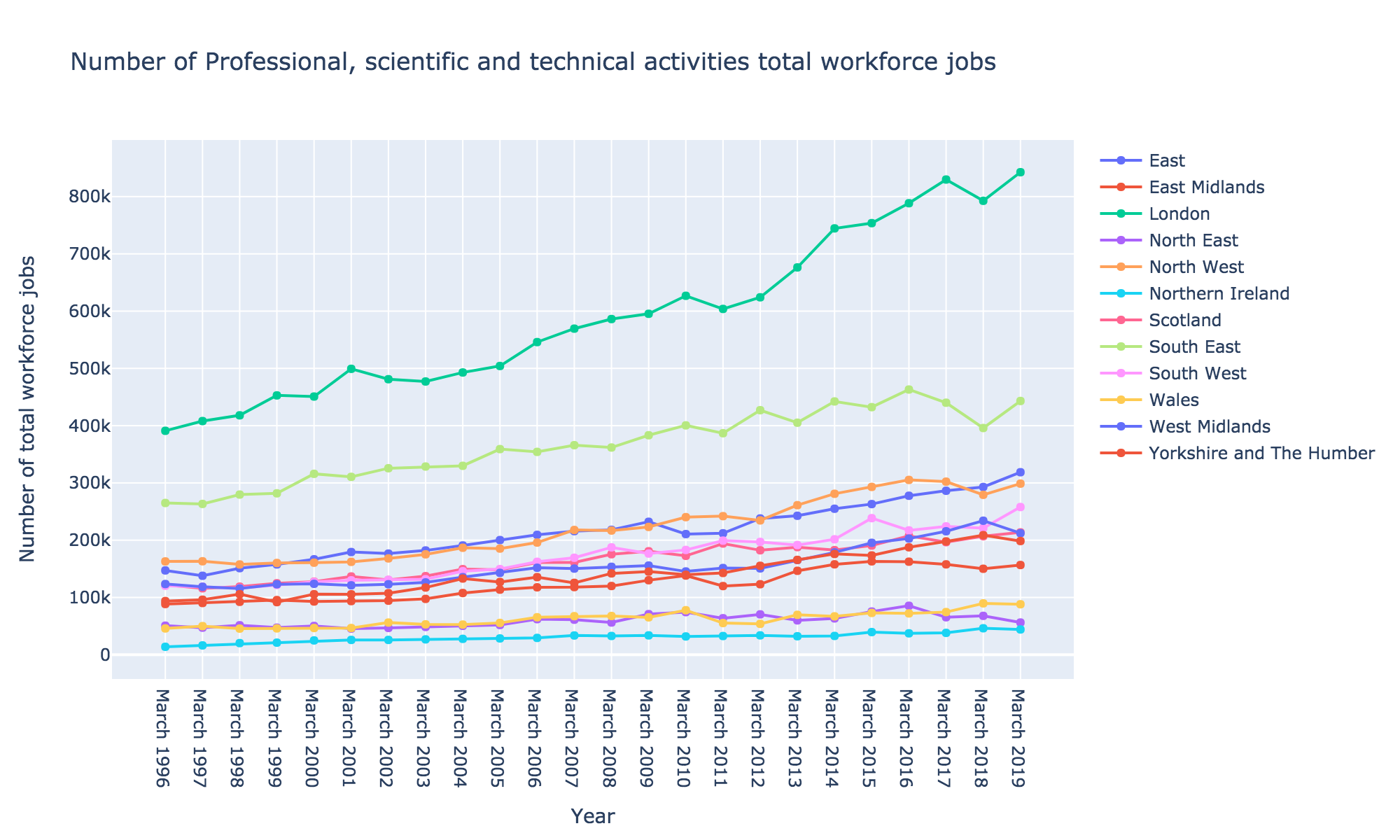Blog for CodersVisualization of workforce jobs for professional, scientific and technical activities across regions¶

In the second episode of data visualization for everyone series, we'll use Plot.ly for Python visualization library. Plot.ly provides interactive and nice-looking visualizations for Python, MATLAB, R, and JS. We'll use Python for the rest of the notebook, to be consistent with previous episode.

We'll visualize number of jobs across regions in UK, specifically Professional, scientific, and technical activity jobs. You can download this data or similar statistics from here.

We cleaned up the header from the data and for the future reference, the header is as follows:

Workforce jobs by industry (SIC 2007) - seasonally adjusted
Analysis   :    standard
Industry   :    M : Professional, scientific and technical activities
Item Name  :    total workforce jobs
Reference  :    https://www.nomisweb.co.uk/query/construct/summary.asp?mode=construct&version=0&dataset=130

You can download cleaned-up data in a CSV format, which we'll be using throughout this notebook here.

First, we need to read the CSV file, using the pandas library, just like we did on the previous notebook.

In :
import pandas as pd

Let's preview the data.

In :
df.tail()
Out:
Date East East Midlands London North East North West Northern Ireland Scotland South East South West Wales West Midlands Yorkshire and The Humber
19 March 2015 263044 163039 753700 75443 293052 39562 190611 432329 238520 72977 195420 173415
20 March 2016 277624 162464 788461 85517 305256 37344 207532 463102 217122 72170 202833 187615
21 March 2017 286329 157806 829812 65403 302250 38155 196561 440078 224186 74490 215548 197930
22 March 2018 292895 150110 792677 67874 279243 46213 206881 395901 221016 89701 234002 208884
23 March 2019 318687 156671 842619 56237 298771 44113 213631 443117 257923 88120 212209 198537

Import the plotly library and initialize for using with jupyter notebook.

In :
from plotly.offline import iplot, init_notebook_mode
import plotly.graph_objects as go

init_notebook_mode(connected=True)

Visualizing the Data¶

We'll use scatter plot visualization using go.Scatter module.

We'll plot Date column in x axis, and North East column in the y axis.

In :
fig = go.Figure(data=go.Scatter(x=df["Date"], y=df["North East"]))
iplot(fig)

Let's add circle markers on the line to improve the appearance of the visualization. To do that, we can specify mode parameter as lines+markers where the previous value was lines as default.

In :
fig = go.Figure(data=go.Scatter(x=df["Date"], y=df["North East"], mode="lines+markers"))
iplot(fig)

Annotate the plot¶

To put text labels alongside the markers, we can change mode parameter to lines+markers+text. That way, we can show whatever we provide in the text parameter. Also, we can specify where the text will be shown with the textposition parameter. If we don't provide the textposition, the deafult parameter will be middle center which will plot on the markers. For the sake of readability, we'll change it to top center. Let's put the number of jobs on text and change mode parameter.

In :
fig = go.Figure(
data=go.Scatter(
x=df["Date"],
y=df["North East"],
text=df["North East"],
mode="lines+markers+text",
textposition="top center",
)
)
iplot(fig)

To annotate some values over the scatter plot, we can update the figure's layout by update_layout method where we can provide annotation property as a list of Annotation objects. For instance, we can annotate the minimum and maximum number of jobs in the North East by creating two annotations as follows.

In :
min_annotation = go.layout.Annotation(
x=df["North East"].idxmin(),  # the index of the minimum value
y=df["North East"].min(),  # minimum value of North East column
text="Minimum",  # annotation text
ay=-70,  # margin for annotation in y-axis
ax=0,  # margin for annotation in x-axis
showarrow=True,  # whether or not showing and arrow head
)

max_annotation = go.layout.Annotation(
x=df["North East"].idxmax(),  # the index of the maximum value
y=df["North East"].max(),  # maximum value of North East column
text="Maximum",  # annotation text
ay=-40,  # margin for annotation in y-axis
ax=0,  # margin for annotation in x-axis
showarrow=True,  # whether or not showing and arrow head
)

fig.update_layout(annotations=[min_annotation , max_annotation])
iplot(fig)
In [ ]:
min_annotation = go.layout.Annotation(
x=df["North East"].idxmin(),  # returns the index with of the minimum value
y=df["North East"].min(),  # returns the minimum value
text="Minimum Value",
showarrow=True,
ay=-100,  # margin in y axis for the annotation
)

max_annotation = go.layout.Annotation(
x=df["North East"].idxmax(),  # returns the index with of the maximum value
y=df["North East"].max(),  # returns the maximum value
text="Maximum Value",
showarrow=True,
ay=-60,  # margin in y axis for the annotation
)
fig = go.Figure(
data=go.Scatter(
x=df["Date"],
y=df["North East"],
text=df["North East"],
mode="lines+markers+text",
textposition="top center",
),
layout=go.Layout(annotations=[min_annotation, max_annotation]),
)

iplot(fig)

Let's add a title and axis labels to our plot. We can either update the layout of the figure, just like we did above, or we can specify layout property when we define the Figure object, like below:

In :
fig = go.Figure(
data=go.Scatter(
x=df["Date"], y=df["North East"], text=df["North East"], mode="lines+markers"
),
layout=go.Layout(
title="Number of Professional, scientific and technical activities total workforce jobs",
xaxis_title="Year",
yaxis_title="Number of total workforce jobs",
),
)
iplot(fig)
In :
# note that we don't provide anything to the data property. We'll add them later.
fig = go.Figure(
layout=go.Layout(
title="Number of Professional, scientific and technical activities total workforce jobs",
xaxis_title="Year",
yaxis_title="Number of total workforce jobs",
width=1000,
height=600
)
)

for col in df.columns[1:]:  # here col represents the column name